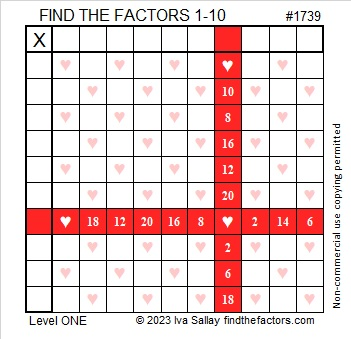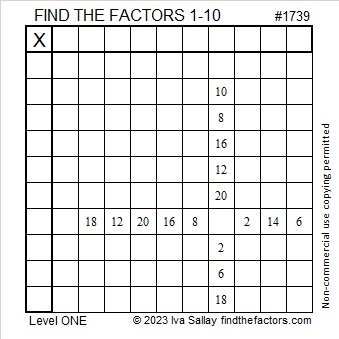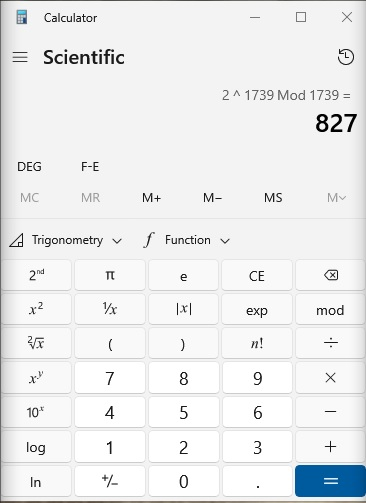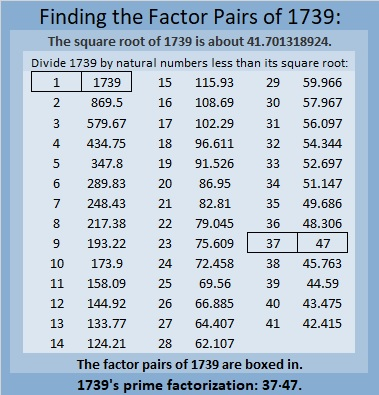# 1739 A Gift for a Valentine

Contents

### Today’s Puzzle:

Here’s a level 1 Valentine’s gift puzzle to start off the month of February. Place the numbers 1 to 10 in both the first column and the top row so that those numbers are the factors of the given number clues.And here’s a heartless copy of the same puzzle that may be more printer-friendly.### Factors of 1739:

Is 1739 a prime number? It isn’t divisible by 2 or 5.
1 + 7 + 3 + 9 = 20, so it isn’t divisible by 3.
1 – 7 + 3 – 9 = -12, so it isn’t divisible by 11.
√1739 is a little more than 41. Should I try dividing 1739 by every other prime number less than 41? I don’t know divisibility tricks for most of those prime numbers!

Here’s a shortcut I first wrote about in 341 is the smallest composite number that gives a false positive for this Quick Prime Number Test: First I type into my computer’s scientific calculator:
2  xʸ  1739  Mod  1739  =

If the answer on the calculator is anything other than “2”, then it can’t be a prime number. If it is “2”, it very likely is prime. As you can see by the screenshot of my calculator, 1739 is not prime!• 1739 is a composite number.
• Prime factorization: 1739 = 37 × 47.
• 1739 has no exponents greater than 1 in its prime factorization, so √1739 cannot be simplified.
• The exponents in the prime factorization are 1 and 1. Adding one to each exponent and multiplying we get (1 + 1)(1 + 1) = 2 × 2 = 4. Therefore 1739 has exactly 4 factors.
• The factors of 1739 are outlined with their factor pair partners in the graphic below.### More About the number 1739:

1739 is the difference of two squares in two ways:
870² – 869² = 1739, and
42² – 5² = 1739.

That means 1739 is only 25 numbers away from the next perfect square!

This site uses Akismet to reduce spam. Learn how your comment data is processed.